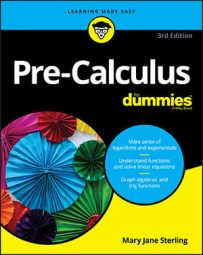##### Pre-Calculus For DummiesEach conic section has its own standard form of an equation with x- and y-variables that you can graph on the coordinate plane. You can write the equation of a conic section if you are given key points on the graph.

Being able to identify which conic section is which by just the equation is important because sometimes that's all you're given (you won't always be told what type of curve you're graphing). Certain key points are common to all conics (vertices, foci, and axes, to name a few), so you start by plotting these key points and then identifying what kind of curve they form.

The equations of conic sections are very important because they tell you not only which conic section you should be graphing but also what the graph should look like. The appearance of each conic section has trends based on the values of the constants in the equation. Usually these constants are referred to as a, b, h, v, f, and d. Not every conic has all these constants, but conics that do have them are affected in the same way by changes in the same constant. Conic sections can come in all different shapes and sizes: big, small, fat, skinny, vertical, horizontal, and more. The constants listed above are the culprits of these changes.

An equation has to have x2 and/or y2 to create a conic. If neither x nor y is squared, then the equation is that of a line. None of the variables of a conic section may be raised to any power other than one or two.

Certain characteristics are unique to each type of conic and hint to you which of the conic sections you're graphing. In order to recognize these characteristics, the x2 term and the y2 term must be on the same side of the equal sign. If they are, then these characteristics are as follows:
• Circle: When x and y are both squared and the coefficients on them are the same — including the sign.

For example, take a look at 3x2 – 12x + 3y2 = 2. Notice that the x2 and y2 have the same coefficient (positive 3). That info is all you need to recognize that you're working with a circle.

• Parabola: When either x or y is squared — not both.

The equations y = x2 – 4 and x = 2y2 – 3y + 10 are both parabolas. In the first equation, you see an x2 but no y2, and in the second equation, you see a y2 but no x2. Nothing else matters — signs and coefficients change the physical appearance of the parabola (which way it opens or how wide it is) but don't change the fact that it's a parabola.

• Ellipse: When x and y are both squared and the coefficients are positive but different.

The equation 3x2 – 9x + 2y2 + 10y – 6 = 0 is one example of an ellipse. The coefficients of x2 and y2 are different, but both are positive.

• Hyperbola: When x and y are both squared, and exactly one of the coefficients is negative and exactly one of the coefficients is positive.

The equation 4y2 – 10y – 3x2 = 12 is an example of a hyperbola. This time, the coefficients of x2 and y2 are different, but exactly one of them is negative and one is positive, which is a requirement for the equation to be the graph of a hyperbola.